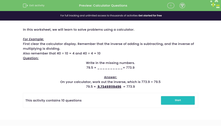# Use a Calculator to Solve a Variety of Problems

In this worksheet, students will solve questions using a calculator.Key stage:  KS 3

Curriculum topic:   Number

Curriculum subtopic:   Use Calculators/Technology for Accuracy

Difficulty level:#### Worksheet Overview

In this activity, we will learn to solve problems using a calculator.

Let's say we wanted to find the missing value in the following calculation:

79.5 × __________= 773.9

We need to do the inverse of multiplication, which is division.

We need to do 773.9 ÷ 79.5

Clearly, a calculator is called for here!First of all, clear the calculator display of any previous calculations.

Now enter: 773.9 ÷ 79.5 =

It should come up with the answer 9.734591195 on the screen.

We usually round numbers to one decimal place unless asked to do something different, but sometimes we round to three decimal places, to be more accurate.

In this case, we would put 9.735 as the answer.

Now we can fill in the missing number:  79.5 × 9.735 = 773.9

We might have to do percentages on the calculator. Don't worry if your calculator doesn't have a % sign - many don't!

Let's say we were asked to find 59% of 180

A percentage simply means that number divided by 100, so we can change 59% into 0.59 by dividing by 100.

Then we simply do 0.59 x 180 =

We find that 106.2 is our answer.

Let's have a go at some questions now.

### What is EdPlace?

We're your National Curriculum aligned online education content provider helping each child succeed in English, maths and science from year 1 to GCSE. With an EdPlace account you’ll be able to track and measure progress, helping each child achieve their best. We build confidence and attainment by personalising each child’s learning at a level that suits them.

Get started